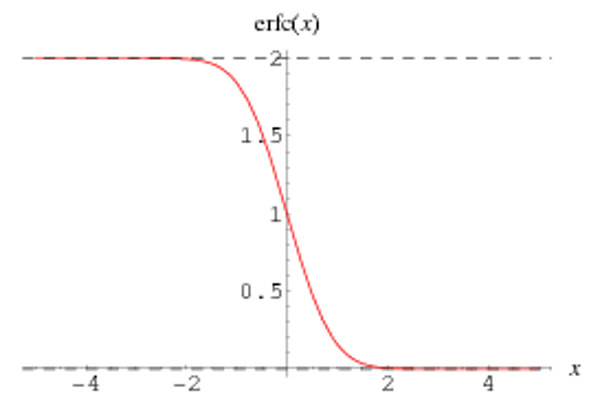Trusted answers to developer questions
Trusted Answers to Developer Questions

Related Tags

c

# What is erfcf in C?

Hassaan Waqar

Grokking Modern System Design Interview for Engineers & Managers

Ace your System Design Interview and take your career to the next level. Learn to handle the design of applications like Netflix, Quora, Facebook, Uber, and many more in a 45-min interview. Learn the RESHADED framework for architecting web-scale applications by determining requirements, constraints, and assumptions before diving into a step-by-step design process.

The erfcf function is defined in the math.h header file in C. It takes in a single parameter, a float value, and then computes the complementary error function of the given argument, returning a type float value.

The complementary error function in mathematics is defined as follows:

erfc $z$ = $1- \frac{2}{\sqrt{\pi}}$ $\int_{0}^{arg} e^{-t^2} dt$

= $\frac{2}{\sqrt{\pi}}$ $\int_{arg}^{\infty} e^{-t^2} dt$

The argument to the erfcf function serves as the lower limit in the above integral.

The error function is most often used in probability and statistics. It integrates the normal distribution and gives the probability that a normally distributed random variable Y (with mean 0 and variance ½) falls into the range [−x, x].

The complementary error function, combined with its series expansion, provides approximate expressions for small and large values of x.

The erfc function in mathematics caters to values of all data types. There is a unique function for each data type in C.

The figure below shows the plot of the erfc function:erfc function [Image taken from WolframAlpha]

The illustration below shows how erfcf function works:

How does erfcf work?

## Declaration

The erfcf function is defined as follows:

float erfcf(float arg);


It takes in a single value of type float and computes its complementary error function. It then returns the answer of type float.

## Error Handling

The erfcf function returns special values for certain arguments:

Special Value Returned
+INFINITY +0
-INFINITY +2
NAN NAN

## Examples

The following code snippet shows how we can use the erfcf function:

#include <stdio.h> // Including header file for printf function#include <math.h>  // Including header file for erfcf functionint main (){  float param, result;  param = 1.5;  result = erfcf(param);  printf("erfcf (%f) = %f\n", param, result);  return 0;}

The following code snippet shows how error handling in the erfcf function works:

#include <stdio.h> // Including header file for printf function#include <math.h>  // Including header file for erfcf functionint main (){  printf("erfcf (%f) = %f\n", INFINITY, erfcf(INFINITY));  printf("erfcf (%f) = %f\n", -INFINITY, erfcf(-INFINITY));  return 0;}

RELATED TAGS

c

CONTRIBUTOR

Hassaan Waqar